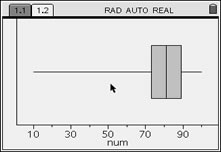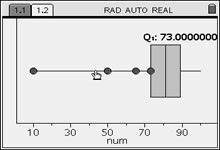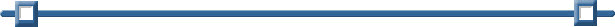Box-and-Whisker Plots

Given the data set
{85, 100, 97, 84, 73, 89, 73, 65, 50, 83, 79, 92, 78, 10},
create a box and whisker plot to represent this data.

There are two forms of box-and-whisker plots -- standard box-and-whisker and modified.

The standard box-and whisker plot includes ALL data points, including what are called outliers. Outliers are points that are far left or far right of the data set and may detract from a representative picture of the data. Outliers are points that are 1.5*IQR beyond the quartiles. (IQR stands for the Interquartile Range which is Q3 – Q1.)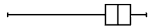Standard box plot.
The modified box-and-whisker plot will not plot outliers as part of the box-and-whisker. The outliers are plotted as individual points beyond the whisker in an attempt to give a more accurate picture of the dispersion of the data.  The modified style is the default on this calculator.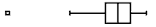Modified box plot.

These two plots represent
the same set of data.

1.  Enter the data on a List & Spreadsheet Page.
This list is named "num".

(See Lists and Spreadsheets for entering data.)2.  Create a Data & Statistics Page
Press#5Data & Statistics.

At first, a generic statistics graph will appear.3. Move the cursor to hover over the Click to add variable at the bottom of the screen. Click. Choose num from the choices.

A dot plot will appear.4.  Pressand change the graph type to Box Plot.

This is a modified box-and-whisker plot with the outlier separated from the rest of the data display.

Note: If there are no points in your data outside of 1.5 * Interquartile Range, no outliers will be displayed.5. As you move the cursor to hover over the box-and-whisker plot, you will see the values for the minimum (not including outlier), first quartile, median, third quartile, and the maximum.

If you move your cursor on the outlier and press Click, you will see its value.6. Creating a standard boxplot
You can create a standard box-and-whisker plot by extending the whiskers of the modified box plot. Remember, in a standard box-and-whisker plot, the whiskers are determined by plotting the minimum and maximum values, with no attention given to outliers.

Press MENU, #2 Plot Properties, #3 Extend Box Plot Whiskers.
Hovering will now show the minimum box value as 10.